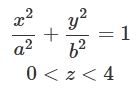The Geometry of Space: Definition, Uses, and Examples

Instructor: Yuanxin (Amy) Yang Alcocer

Amy has a master's degree in secondary education and has been teaching math for over 9 years. Amy has worked with students at all levels from those with special needs to those that are gifted.

Knowing the geometry of objects and things in the real world is a very useful skill. Architects use geometry to aid them in designing buildings and cities that work. Engineers use geometry to build working components in a bigger machine.

Using Space

Look around at the different ways that companies package their products. Some companies package their products so they can maximize the number of products that fit into a certain space, while others package their products so they are very bulky. You know how sometimes you have a big box and you think there's something big inside, but when you open it, you find it has lots of packaging and a tiny actual product inside? This is where stores like Costco and IKEA are masters at using the geometry of space to maximize the number of items a particular space can hold.

The geometry of space is so much more than mathematical calculations. The geometry of space is about how everything fits together. For example, if you have a packing box, it is the geometry of space that determines just how many items can fit inside the box. It is also the geometry of space that lets you fit more items in a box if they are placed in a certain way.

Definition of Geometry of Space

So what is this geometry of space? It involves three dimensions: a length, a width, and a height. Every single object in space has all three of these dimensions. But not all objects are simple block shapes with just one length, one width, and one height. Some objects vary in length, width, and height in different parts of the object. For example, the honeycomb pattern of a beehive has different lengths at different parts of the hexagon that is each honey-containing hole.

To define objects in space, all three dimensions are used. For simple block shapes, a simple l × w × h description is all that is needed. For more complicated objects, more complex mathematical functions are used. For example, a sphere can be written mathematically with this function.The height of the cylinder can be defined by setting limits to the z variable. For this example, the cylinder has a height of 4. The x, y, and z variables represent each of the three dimensions in space. The x and y variables typically represent the length and width of objects, while the z variable represents the height of the object.

Using the Geometry of Space

Any problem that requires calculating space uses the geometry of space. For example, problems that ask you to find out how many packages fit inside another larger package use the geometry of space. Toys that have moving parts that allow you to turn the toy from a box shape into a robot use geometry of space to figure out where and how the parts need to fit together to get from one shape to another.

Example

Let's look at an example problem.

To unlock this lesson you must be a Study.com Member.

Register to view this lesson

Are you a student or a teacher?